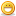menu

# Thread: [Request] Lt.Vachem Bot AutoIt or AHK

1. ## [Request] Lt.Vachem Bot AutoIt or AHK

Hi everyone, i'm looking for a bot to farm LT Vachem, I don't have enough money to buy DemonBuddy also I need a good bot running with AutoIt or AHKI'm able to give a few € if it's a homemade (working) botThanks a lot, you're a great community !Reply With Quote

2. (herp derp)Reply With Quote

3. I farm with during 20min and I take 2 level (Monk- Normal SoftCore), I don't think it's nerfedReply With Quote

4. Your inbox was full so I'll post in here.

Originally Posted by Ponoir
In Normal Mode with 21% gems on hat ~7Kxp/run (5600 by the quest rewrd +500gold)
Then why not do ZK? It gives 10k XP in normal (20k in hell), there is many scripts here... Btw gem on hat doesn't give extra XP for the quest, only for killing mobs.Reply With Quote

5. I'll write a bot for a few euros, I'm USD though so I'll post a paypal donation thing. I need your character build / skills and resolution you would like.Reply With Quote

6. I have a crude hacked version I have been working on right now but im no damn coder thats for sure but the one I have done works perfect for my monk, but again its seriously hacked to work lol..

here is the main run script i have been working on for it

Code:
``` Func RestartRun()
While \$go
if(\$Begin) Then
\$i = 0
Sleep(1000) ;had to add this since the icon doesn't show up RIGHT away in game
MouseClick("left", Round(230*\$x_ratio),Round(484*\$y_ratio))
Sleep(240)
Mousemove (Round(819*\$x_ratio),Round(265*\$y_ratio),10)
Mousedown("left")
Sleep(1300)
mouseup ("left")
mouseclick("left", Round(566*\$x_ratio),Round(318*\$y_ratio))
sleep(100)
Mousemove(Round(819*\$x_ratio),Round(296*\$y_ratio))
Sleep(240)
mousedown("left")
Sleep(240)
Mousemove(Round(819*\$x_ratio),Round(516*\$y_ratio), 10)
sleep(240)
mouseup ("left")
sleep(100)
MouseClick("left", Round(539*\$x_ratio),Round(516*\$y_ratio))
sleep(240)
MouseClick("left", Round(539*\$x_ratio),Round(516*\$y_ratio))
sleep(240)
MouseClick("left", Round(1200*\$x_ratio),Round(865*\$y_ratio))
sleep(240)
MouseClick("left", Round(847*\$x_ratio),Round(632*\$y_ratio))
sleep(240)
MouseClick("left", Round(238*\$x_ratio),Round(419*\$y_ratio))

MouseClick("left", Round(567* \$x_ratio),Round(495 * \$y_ratio)) ;starts the main run
Sleep(1800)
MouseClick("left", Round(238*\$x_ratio),Round(384*\$y_ratio))
Sleep(2100)
MouseClick("left", Round(1052*\$x_ratio),Round(16*\$y_ratio))
Sleep(3200)
MouseClick("left", Round(1532*\$x_ratio),Round(310*\$y_ratio))
Sleep(2000)
MouseClick("left", Round(1671*\$x_ratio),Round(986*\$y_ratio))
sleep(1800)
MouseClick("left", Round(1499*\$x_ratio),Round(941*\$y_ratio))
sleep(1800)
MouseClick("left", Round(1267*\$x_ratio),Round(956*\$y_ratio))
sleep(1800)
MouseClick("left", Round(976*\$x_ratio),Round(570*\$y_ratio))
sleep(300)
MouseClick("left", Round(1056*\$x_ratio),Round(480*\$y_ratio))
sleep(280)
send ("{esc}")
sleep(120)
MouseClick("left", Round(1710*\$x_ratio),Round(350*\$y_ratio))
Sleep(2000)

Sleep(280)
MouseClick("left",Round(1704*\$x_ratio),Round(1000*\$y_ratio)) ;move into position to attack
Sleep(2800)
Send("{space}")
sleep(240)
Send("{space}")
sleep(240)
send("3")
sleep(5000)
send("1")
Sleep(500)
sleep(2000)
send("{shiftdown}")
sleep(50)
Send("{2 DOWN}")
sleep(4000)
send("{2 Up}")
sleep(50)
send("{shiftup}")
sleep(400)
If Not \$Logout And Not \$Dead And Not \$Window Then
MouseClick("left",Round(1399*\$x_ratio),Round(826*\$y_ratio))	;click on cage to open it
Sleep(\$walktime)
sleep(1500)
send("{space}")
sleep(80)
send("{space}")
sleep(80)
send("{space}")
sleep(80)
MouseClick("left",Round(257*\$x_ratio),Round(21*\$y_ratio)) ;click to get out of cellar
Sleep(\$walktime)
MouseClick("left",Round(905*\$x_ratio),Round(335*\$y_ratio))
MouseClick("left",Round(272*\$x_ratio),Round(243*\$y_ratio)) ;move to attack outside cellar
sleep(\$walktime)
send("{SHIFTDOWN}")
MouseClick("left",Round(947*\$x_ratio),Round(523*\$y_ratio)) ; attacks
send ("{SHIFTUP}")
sleep(80)
send ("{SHIFTDOWN}")
MouseClick("left",Round(947*\$x_ratio),Round(523*\$y_ratio)) ;attacks
send ("{SHIFTUP}")
sleep(100)
send ("{shiftdown}")
sleep(40)
Send ("{2 DOWN}")
sleep (6000)
send ("{2 Up}")
send ("{shiftup}")
MouseClick("left",Round(1058*\$x_ratio),Round(377*\$y_ratio))
sleep(500)
MouseClick("left",Round(790*\$x_ratio),Round(574*\$y_ratio))
sleep(500)
MouseClick("left",Round(1172*\$x_ratio),Round(637*\$y_ratio))
sleep(3000)
send("{esc}")
If Not \$Logout And Not \$Dead And Not \$Window Then
Sleep(700)
Send("t")
Sleep(\$tptime)
Sleep(2000)
If Not \$Logout And Not \$Dead And Not \$Window Then
Sleep(300)
Sleep(200)
MouseClick("left", Round(956*\$x_ratio),Round(579*\$y_ratio)) ;button to leave game

EndIf
EndIf
EndIf
EndIf
WEnd
Call("RestartRun")
EndFunc```
again like I said im no damn coder, so getting this working right and how it works for me is 2 different things lol. it work for me and my system as long as it doesnt hiccup or studder.

if someone wants to work on it and get it working how it should that would be awesome.

the first part is for changing the mission, and it works flawlessly since it cant just resume it has to make the actual change the the map before entering.Reply With Quote

7. Your inbox was full so I'll post in here.

Then why not do ZK? It gives 10k XP in normal (20k in hell), there is many scripts here... Btw gem on hat doesn't give extra XP for the quest, only for killing mobs.
Isn't ZK really only useful if you are running 2 accounts on it? Perhaps he only has one, or perhaps he's trying to avoid the popular area for fear of exploit banning.Reply With Quote

8. @FattyXP Yepi don't want to be in the mass of maasive ban account !Reply With Quote